# Principal ideal ring

(diff) ← Older revision | Latest revision (diff) | Newer revision → (diff)
Jump to: navigation, search

An associative ringwith a unit element (cf. Associative rings and algebras) in which all right and left ideals are principal, i.e. have the formand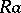, respectively, where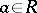. Examples of principal ideal rings include the ring of integers, the ring of polynomialsover a field, the ring of skew polynomials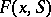over a fieldwith an automorphism(the elements ofhave the form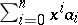,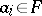, the addition of these elements is as usual, while their multiplication is defined by the associativity and distributivity laws and by the equation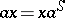where), the ring of differential polynomialsover a fieldwith a derivation(this ring also consists of the elements,; addition is carried out in the ordinary way while multiplication is determined by the equation,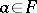). A principal ideal ring without a zero divisor is called a principal ideal domain. A commutative principal ideal ring is a direct sum of principal ideal domains and a principal ideal ring with a unique nilpotent prime ideal (cf. Nilpotent ideal; Prime ideal). Ifis a principal ideal domain, then two non-zero elementsandofhave a greatest common left divisor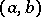and a least common right multiple, which are defined as the elements that satisfy the equations: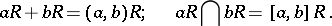The elementsandare unique, up to an invertible right factor. A principal ideal domain is a unique factorization domain. The two-sided ideals of a principal ideal domain form a free commutative multiplicative semi-group with a zero and a unit element (the maximal ideals of the ring are the free generators of this semi-group).

A submoduleof a free moduleof finite rankoveris a free module of rank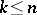over, and in the modulesandit is possible to select bases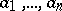and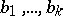so that,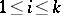, where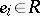andis a complete divisor, i.e.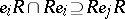, of the elements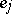if. Each finitely-generated moduleoveris a direct sum of cyclic modules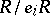,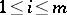, whereand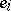is a complete divisor ofif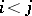,. This theorem generalizes the fundamental theorem on finitely-generated Abelian groups (cf. Abelian group). The elements,, in the preceding theorem are unambiguously defined up to a similarity (cf. Associative rings and algebras). These elements are called invariant factors of. Moreover,can be represented as a direct sum of indecomposable cyclic modules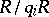, where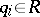,. The elements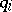,, are defined up to a similarity, and are called elementary divisors of the module. If the principal ideal domainis commutative, then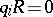or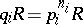,, where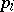are irreducible (prime) elements of. The ordinary properties of elementary divisors and invariant factors of linear transformations of finite-dimensional vector spaces follow from the above statements .

How to Cite This Entry:
Principal ideal ring. Encyclopedia of Mathematics. URL: http://encyclopediaofmath.org/index.php?title=Principal_ideal_ring&oldid=14409
This article was adapted from an original article by L.A. Bokut' (originator), which appeared in Encyclopedia of Mathematics - ISBN 1402006098. See original article